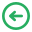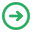# NCERT Solutions for Class 10 Maths Chapter 12 Free PDF DownloadTable of content

## NCERT Solutions for Class 10 Maths Chapter 12 – Areas Related to Circle

First of all, NCERT solutions for class 10 maths Chapter 12 is vital for class 10m students. These solutions are very useful for students preparing for class 10 Board examination. NCERT provides solutions to important questions from all topics of Maths. They present difficult information in a simple manner before the students.

NCERT solutions help in reducing the stress of students. Furthermore, they help in saving a lot of time for students. A student can clarify any doubt he has in Maths from these solutions. These solutions are a great help in getting excellent marks in board examination. Toppr has gained the reputation of mastering NCERT solutions. Hence, it is recommended for students to click on Toppr links below for NCERT solutions.

### CBSE Class 10 Maths Chapter 12 – Areas Related to Circle NCERT Solutions

A circle refers to a simple closed shape. It has points on a plane which exist at a constant distance from the center. Area of a circle = πr2, where the r= radius and π= is a constant whose value is 3.14.

NCERT solutions for class 10 maths chapter 12 deals with areas related to circles. Most noteworthy, in this chapter students, will learn the area of the circle. NCERT solutions for class 10 maths chapter 12 cover more than 30 questions. This chapter begins with an analysis of the perimeter and area of the circle. The application of this knowledge happens to find areas of two special parts. These special parts of a circular region are sector and segment.### Sub-topics covered under NCERT Solutions for Class 10 Maths Chapter 12

• 12.1 Introduction
• 12.2 Perimeter and Area of a Circle- A Review
• 12.3 Areas of Sector and Segment of a Circle
• 12.4 Areas of Combinations of Plane Figures
• 12.5 Summary

### NCERT Solutions for Class 10 Maths Chapter 12

Students in Class 10 are probably well aware of calculations of plane figures. This chapter explores the topic of Circles in more detail. The calculations of Circle are more complex in contrast to previous classes. This chapter deals specifically with areas to circles.

The explanation of various sub-topics is below:

Introduction– First of all, this part introduces students with areas related to circles.

Perimeter and Area of a Circle– A review– Here calculations are present. These calculations relate to the Perimeter and Area of a Circle. Area of a Circle = πr2 while, Perimeter of a Circle = 2πr.

Areas of Sector and Segment of a Circle– First of all, the Sector of Circle is a portion of a circle. Area of a sector of a Circle is 1/2 r2θ, where r = radius, θ = angle in radians subtended by an arc at the center of the circle. The segment of a Circle is a region of the circle which is cut-off from the rest. The area of Segment is equal to the area of the circular sector minus the area of the triangular portion. Furthermore, detailed information is present in this sub-topic.

Areas of combinations of plane figures– The Plane figure is a closed, two-dimensional figure. Hence, it is also called as a flat figure. A Circle can probably have two or more plane figures. So this sub-unit deals with areas of plane figures.

Summary– Finally, a summary of all previous information to mark the end of this chapter.

You can download NCERT Solutions for Class 10 Maths Chapter 12 belowDownload the Toppr app. This will certainly be of big help to students. The app is beneficial for students aspiring to appear in important examinations. Some important features of Toppr are below:

• Huge variety of content,
• High success ratio,
• Accurate content,
• An easy search feature,
•  Expert content.

Share with friendsCustomize your course in 30 seconds

Which class are you in?

5th
6th
7th
8th
9th
10th
11th
12th
Get ready for all-new Live Classes!
Now learn Live with India's best teachers. Join courses with the best schedule and enjoy fun and interactive classes.Ashhar Firdausi
IIT Roorkee
BiologyDr. Nazma Shaik
VTU
ChemistryGaurav Tiwari
APJAKTU
Physics
Get Started
Customize your course in 30 seconds

Which class are you in?

No thanks.